TitleIntermediate Algebra
Answer/Discussion to Practice Problems
Tutorial 2: Algebraic ExpressionsAnswer/Discussion to 1a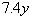when y = 2.2 In this problem we will be replacing y with 2.2 and then multiply: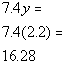*Replace y with 2.2 *(  ) is another way to write multiplication *Multiply numbers togetherAnswer/Discussion to 1b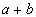when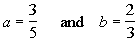In this problem, we will replacing a with 3/5 and b with 2/3 and then adding the two together: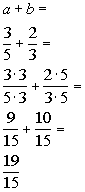*Replace a with 3/5 and b with 2/3 *Mult. top and bottom of 1st fraction by 3 to get LCD of 15 *Mult. top and bottom of 2nd fraction by 5 to get LCD of 15     *Add the fractions together

 Note that if you need to review adding fractions go to this link: fractions (return to problem 1b)Answer/Discussion to 1c  You need to know how much area of carpet to purchase to carpet your rectangular floor.  You will need to use the algebraic expression lw to find it's area, where l represents the length of the rectangular floor and w is the width of it.  If your rectangular floor is 15 feet long and 8 feet wide, find how much area of carpet you would need to cover it. In this problem we will be replacing l with 15 and w with 8 and multiplying the two together: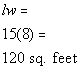*Replace l with 15 and w with 8 *Multiply the two numbers togetherAnswer/Discussion to 2a 15 more than a number.   In this problem what mathematical operation would we substitute the phrase 'more than' with?  I hope you said addition. Once again, we have an unknown number so we will replace the phrase 'a number' with the variable x. Putting it all together we get:

 15 more than a number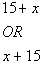* 'more than' = + *'a number' = variable xAnswer/Discussion to 2b The quotient of 7 and the product of 3 and a number.     First of all the term quotient is going to be replaced with what mathematical operation?  If you said division you are correct!! Note how 7 immediately follows the phrase the quotient of, this means that 7 is going to be in the numerator.  The phrase that immediately follows the word quotient is going to be in the numerator of it. After the word 'and' you have the phrase the product of 3 and a number.  That is the second part of your quotient which means it will go in the denominator.  And what operation will we have when we do write that product down below?  Multiplication would be the correct response to that.   Let's see what we get when we put all of this together:

 The quotient of 7 and the product of 3 and a number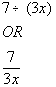*'quotient' = division  *'product' = multiplication  *'a number' = variable xAnswer/Discussion to 2c 17 less than the sum of a number and 8.   First of all we have the phrase less than which mathematically translates as what?  If you said subtraction you are doing great!!  Again, you need to be careful with this phrase - it is very tempting to start off with 17 and put your subtraction sign after the 17 - however, think about it, if you want 17 less than something you are 17 below it, in order to be 17 below something you would have to subtract the 17!!!  So you would not have 17 minus but minus 17 as PART of your expression. The other part of the expression involves the phrase 'the sum of', which would be what mathematical operation?   Addition would be the correct response to that. And as always, when we have a phrase like 'a number' where we have a unknown number, we replace that with a variable. Let's see what we have:

 17 less than the sum of a number and 8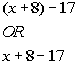*'less than' = - *'sum' = + *'a number' = variable x

Last revised on June 10, 2011 by Kim Seward.
All contents copyright (C) 2002 - 2011, WTAMU and Kim Seward. All rights reserved.